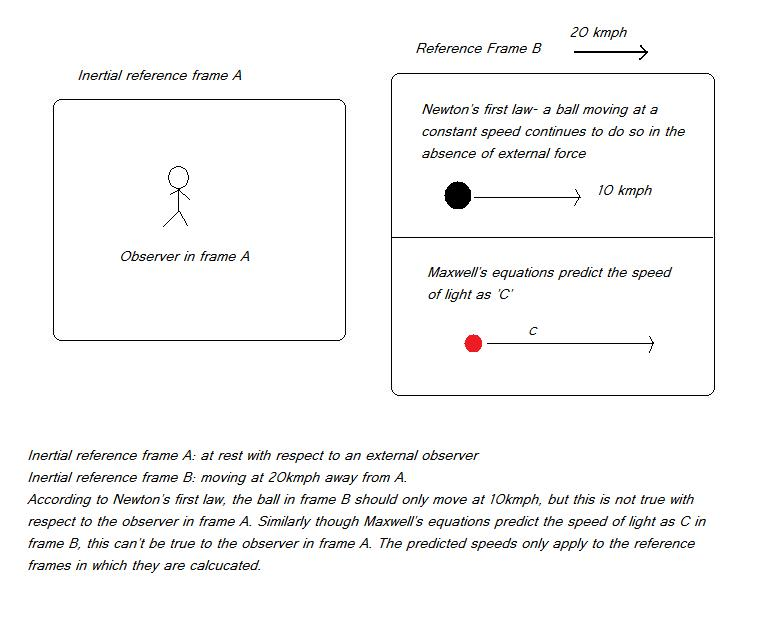## Maxwell’s equations and SOL

Before Einstein, scientists knew that ‘the laws of physics are same in all inertial reference frames’.

From Maxwell’s experiments on electromagnetic fields, it became clear that Light travels at a speed of 3×108 m/sec. Einstein had rightly taken this point and restated the above as “laws of physics including speed of Light (SOL) remain the same in all inertial reference frames”.

Then began all the wrong interpretation-

There wouldn’t have been any confusion if the statement had just meant- ‘Maxwell’s equations predict the same SOL in any inertial reference frame’ but Einstein’s special relativity preaches an entirely weird thing- A flash of light travels at the same speed relative to any observer, irrespective of the motion of the observer or the source.

Though the mistake is immediately evident, it definitely needs a lot more elaboration because the absurd predictions of relativity are deeply engraved into the minds of the scientists and the public.

Let us compare the law of constant speed of light and the Newton’s first Law in two inertial reference frames-In the inertial reference frame B, Newton’s first law predicts that a ball moving at 10kmph continues to move at the same steady velocity in the absence of external force. But it doesn’t say that the ball moves at the same steady velocity of 10kmph with reference to an observer in frame B. Obviously the ball moves at 30kmph according to the observer in frame A.

Similarly, if Maxwell’s equations predict that a flash of light travels at 3×108m/sec in frame B, it doesn’t mean that the light flash travels at the same speed with respect to an observer in frame A.

When a ball’s velocity is not constant to different observers, why should a light photon’s velocity remain the same? Maxwell’s equations didn’t predict that the SOL is constant to differently moving observers.

So there is no reason at all to assume that speed of light is constant to all the observers. Of course this is what commonsense tells us but relativists abandoned this long ago in favour of weird mathematical predications.

•Jerry  On January 8, 2014 at 3:30 am

Of course Maxwell’s equations didn’t predict that the speed of light is constant for all observers—Einstein did. If c is not constant for all observers, then Maxwell’s equations are not invariant, which implies that we could observe absolute motion via optical measurements. However, experimental data is consistent with Einstein’s predictions. Please cite experimental data from the literature that supports your view. Without that, your argument amounts to this: a photon should be no different than a ball (which, of course, is not the refutation of SR your site claims to provide).

•drgsrinivas  On January 11, 2014 at 1:07 am

Experimental data from literature? Any interpretation that goes against the preaching of your relativity religion wouldn’t get published in the journals of your religion. (Of course that’s usually the case with any religious literature!)

Anyhow I don’t blindly believe in the literature, So what I have done is that I have critically examined the experimental data that you all claim as consistent with or proof of your weird theory. To my surprise, relativists have managed to misinterpret even the contradictory data as strong proof of their delusional theory.

•einsteinthezionist  On October 12, 2018 at 3:43 am

Has anyone seriously thought to critically review the validity of the two derived “constants” used in Maxwell’s equations? Mu zero and Epsilon zero seem like OK constants but magnetism is always a property of some matter, if you have no matter then you don’t get any property. If you think you have removed all matter but still have a reading, then either you are seeing instrument error or you should admit that there is a Aether.

Same with Electric charge. Charge is a property, but a property of matter.
Where is the matter that you are saying has the measured value of Epsilon?
Where is the matter that has the property with the measured value of Mu?

Without these two assumptions, irrational assumptions, can Maxwell still derive the speed of light as 300 million meters per second?

Logically, Mu zero and Epsilon zero values should be both set to zero precisely, as a base line for the comparison of different values of matter. How can they have a value? What possesses that measured value? We measured precisely nothing and found that it is three feet long!